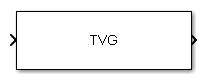# Time Varying Gain

Time varying gain (TVG) control

•Libraries:
Phased Array System Toolbox / Detection

## Description

The Time Varying Gain block applies a time-varying gain to input signals to compensate for geometric range loss at each range gate. Time varying gain (TVG) is sometimes called automatic gain control (AGC).

## Ports

### Input

expand all

Input signal, specified as a vector, matrix, or array.

The size of the first dimension of the input matrix can vary to simulate a changing signal length. A size change can occur, for example, in the case of a pulse waveform with variable pulse repetition frequency.

Data Types: `double` | `single`

Range loss, specified as a column vector. The length of the vector must be equal to or greater than the number of rows in the input signal in Port_1.

The process equalizes power levels across all samples to match a given reference range. The gain is applied to each column in Port_1 independently. The number of rows in Port_1 cannot exceed the length of the loss vector specified in the Range losses (dB) parameter.

#### Dependencies

To enable this port, set Source of range losses parameter to ```Input port```.

Data Types: `double` | `single`

### Output

expand all

Range-compensated signal, returned as a vector, matrix, or array. The range-compensated signal has the same size as the input signal.

Data Types: `double` | `single`

## Parameters

expand all

Source of range loss, specified as `Property` or `Input Port`.

 `Property` Specify range losses using the Range loss (dB) parameter. `Input port` Specify range losses using the input port Port_2 .

Data Types: `char` | `string`

Loss at each input sample range, specified as a vector. Vector elements correspond to the samples in the input signal. Units are in dB.

#### Dependencies

To enable this parameter, set the Source of range losses parameter to `Input port`.

Data Types: `double` | `single`

Loss at the given reference range, specified as a scalar. Units are in dB.

Data Types: `double` | `single`

Block simulation, specified as `Interpreted Execution` or `Code Generation`. If you want your block to use the MATLAB® interpreter, choose `Interpreted Execution`. If you want your block to run as compiled code, choose ```Code Generation```. Compiled code requires time to compile but usually runs faster.

Interpreted execution is useful when you are developing and tuning a model. The block runs the underlying System object™ in MATLAB. You can change and execute your model quickly. When you are satisfied with your results, you can then run the block using ```Code Generation```. Long simulations run faster with generated code than in interpreted execution. You can run repeated executions without recompiling, but if you change any block parameters, then the block automatically recompiles before execution.

This table shows how the Simulate using parameter affects the overall simulation behavior.

When the Simulink® model is in `Accelerator` mode, the block mode specified using Simulate using overrides the simulation mode.

Acceleration Modes

 Block Simulation Simulation Behavior `Normal` `Accelerator` `Rapid Accelerator` `Interpreted Execution` The block executes using the MATLAB interpreter. The block executes using the MATLAB interpreter. Creates a standalone executable from the model. `Code Generation` The block is compiled. All blocks in the model are compiled.

 Block Parameter:`SimulateUsing` Type:enum Values:```Interpreted Execution```, `Code Generation` Default:```Interpreted Execution```